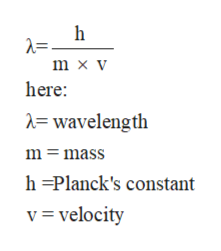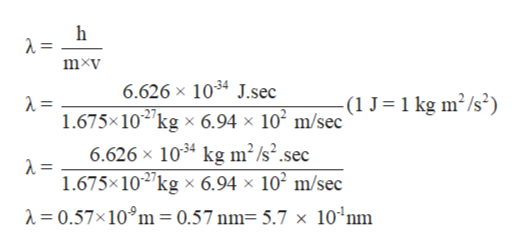# Thermal neutrons are neutrons that move at speeds comparable to those of air molecules at room temperature. These neutrons are most effective in initiating a nuclear chain reaction among 235U isotopes. Calculate the wavelength (in nm) associated with a beam of neutrons moving at 6.94*102 m/s (mass of a neutron=1.675*10-27 kg). Enter your answer in scientific notation.

Question
417 views

Thermal neutrons are neutrons that move at speeds comparable to those of air molecules at room temperature. These neutrons are most effective in initiating a nuclear chain reaction among 235U isotopes. Calculate the wavelength (in nm) associated with a beam of neutrons moving at 6.94*102 m/s (mass of a neutron=1.675*10-27 kg). Enter your answer in scientific notation.

check_circle

Step 1

Electromagnetic spectrum involves different radiations from the lowest to the highest frequency values. Some common radiations which are part of electromagnetic radiations are infrared radiation, visible light, ultraviolet radiation, X-rays, and gamma rays.

Step 2

The de Broglie equation purposed the relation between wavelength and mass of the photon. The mathematical expression for this relation is:help_outlineImage Transcriptioncloseh m x v here: = wavelength m mass h =Planck's constant v= velocity fullscreen
Step 3

Velocity of beam = 6.94*102 m/s

Mass of a neutron=1.675*10-27 kg

Subst...help_outlineImage Transcriptionclosemxv 6.626 x 1034 J.sec -(1 J=1 kg m2/s2) 1.675x102 kg x 6.94 x 102 m/sec 6.626 x 1034 kg m2/s2.sec = 1.675x102kg x 6.94 x 102 m/sec = 0.57x10°m = 0.57 nm= 5.7 x 10°nm fullscreen

### Want to see the full answer?

See Solution

#### Want to see this answer and more?

Solutions are written by subject experts who are available 24/7. Questions are typically answered within 1 hour.*

See Solution
*Response times may vary by subject and question.
Tagged in

### Other